{{announcement.body}}
{{announcement.title}}

# Creating JMeter Variables in Java - The Ultimate Guide

DZone 's Guide to

# Creating JMeter Variables in Java - The Ultimate Guide

### Learn how to create the different types of JMeter variables in Java, details of the different variable types, and how to avoid errors.

· Performance Zone ·
Free Resource

Comment (0)

Save
{{ articles.views | formatCount}} Views

Writing load or functional tests in Apache JMeter™ involves working with different types of variables. Variables have multiple uses, for example, when:

• The API or Web service being tested returns a value or several values and the value data must be compared with the expected result.
• The API or Web service being tested returns a value or several values and the value data must be checked in the database.

Therefore, knowledge and skills of applying variables is the basis for using JMeter. This blog post will explain how to create variables in JMeter when you need to use Java code in your tests.

To create variables in Java language, you can use any standard JMeter element that supports BeanShell. In this blog post, we will use JMeter 3.2 and the JSR223 Sampler element in which Java (BeanShell 2.0b5 / BeanShell Engine 1.0) is selected, as can be seen below: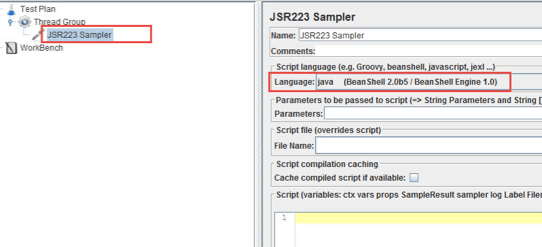Any variable in Java must have a data type. A data type is a characteristic that determines the range of possible values, operations on these values, and how these values are stored in computer memory. Otherwise, the compiler will generate an error.

Java variables support two kinds of data types: simple (also called primitive), and reference data. We will cover both. In the next blog post, we will explain which methods you can use to work with these variables.

JMeter enables writing Java code using a non-strict mode when the data type does not need to be specified, or by strict mode when creating variables (because variables require specifying the data type). The strict mode is fully compatible with the rules for creating and initializing variables in Java. To enable the strict mode you must use the setStrictJava (true) command at the beginning of the script: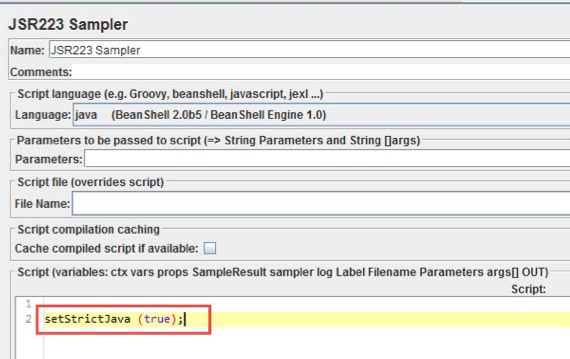There are 8 primitive data types for representing integer, fractional, and boolean values: int, short, long, byte, float, double, char, boolean.

## Variables With an Integer Data Type (int, short, long, and byte)

An integer type is a data type that is used for integer values without a fractional part. The integer type of data includes the following types: int, short, long, and byte. These types allow us to represent both positive and negative values.

You might ask why so many types are available. Why can't just one type be created? The reason is that when creating a variable with a simple data type, a specific amount of computer memory is consumed. So various types are used for the rational use of this memory.

In other words, if the data type int, which occupies 4 bytes of the memory of the computer, is actually suitable for us, and we use the long type, which occupies 8 bytes, then the memory of the computer is inefficiently used. For small software systems such a use would not cause problems, but in large software systems, this can cause memory overflow and software shutdown. In practice, the int data type is often used.

The table below shows the required amount of memory and the range of values allowed for each variable type: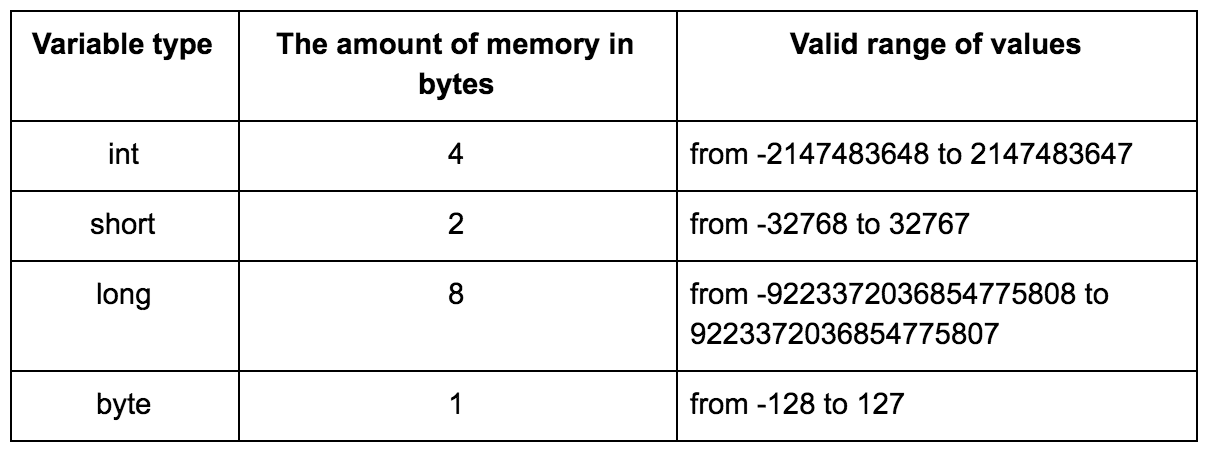An example of creating variables with an integer data type is shown in the image below: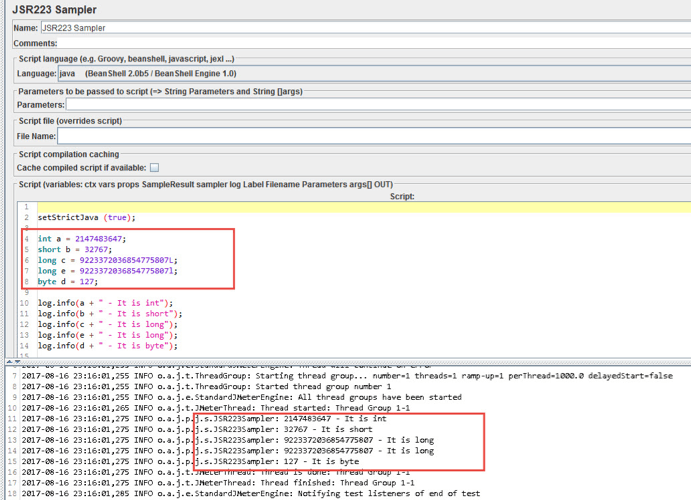This is the example code:

``````setStrictJava (true);

int a = 2147483647;
short b = 32767;
long c = 9223372036854775807L;
byte d = 127;

log.info(a + " - It is int");
log.info(b + " - It is short");
log.info(c + " - It is long");
log.info(d + " - It is byte");``````

Note: When creating a variable with the type long at the end of a numeric value, you must specify l or L. This feature is related to type conversion.

## Numeric Variables With a Floating-point Type (float and double)

Numeric variables with a floating-point type are variables that have integer and fractional parts. These types include float and double. The precision of variables with double type is twice the type of float. The table below shows the required amount of memory and the allowable values for the variable type.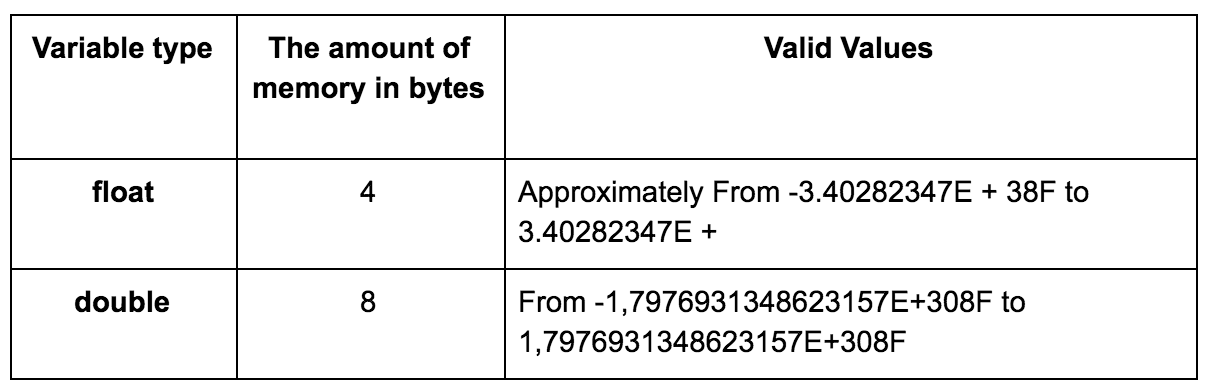An example of creating variables with a simple data type is shown in the image below: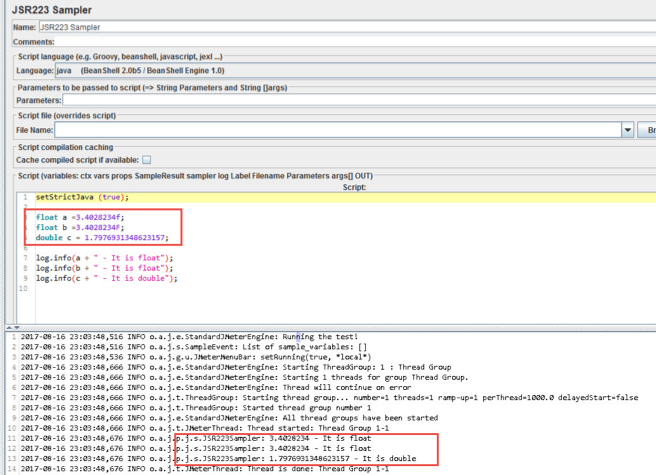This is the example code:

``````setStrictJava (true);

float a =3.4028234f;
float b =3.4028234F;
double c = 1.7976931348623157;

log.info(a + " - It is float");
log.info(b + " - It is float");
log.info(c + " - It is double");``````

Note: When creating a variable with a float type at the end of a numeric value, you must specify f or F. This feature is related to type conversion.

## Variables With the Char Data Type (char)

Variables with the char data type are intended for storing characters in Unicode. This means that you can assign a variable to both a symbol value and the corresponding Unicode numeric value. An example of creating variables with a char type is shown in the image below.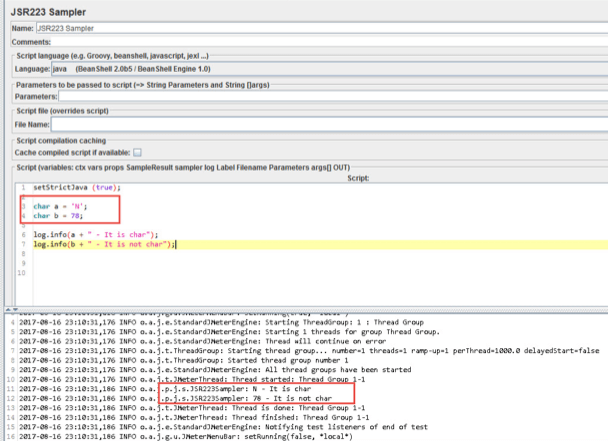This is the example code:

``````setStrictJava (true);

char a = 'N';
char b = 78;

log.info(a + " - It is char");
log.info(b + " - It is not char");``````

Creating a variable with the Char type using the Java language in IntelliJ IDEA: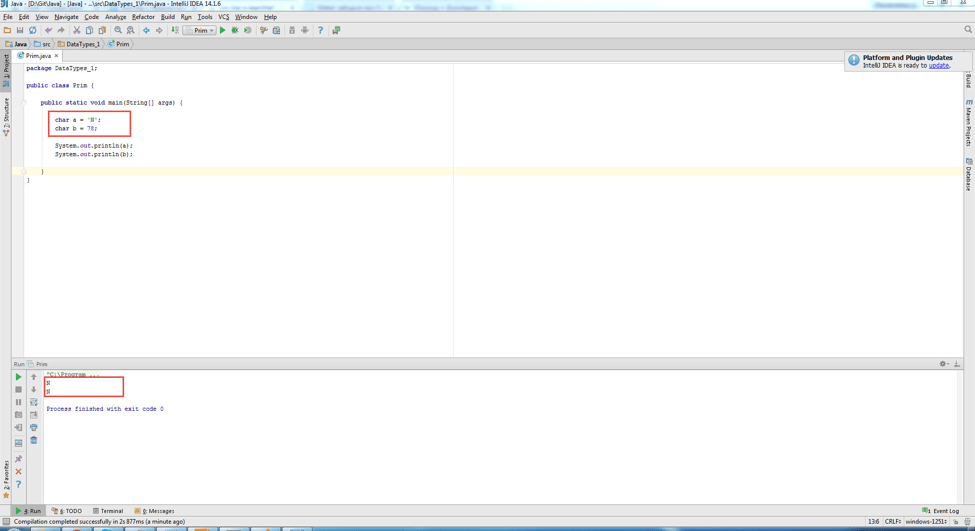Example code in IntelliJ IDEA:

``````public class Prim {
public static void main(String[] args) {

char a = 'N';
char b = 78;

System.out.println(a);
System.out.println(b);

}
}``````

Comparing the images, you can see that in JMeter you cannot create a char type variable by its value in Unicode. Unicode is a character encoding standard. This standard provides a unique code for any character, regardless of platform, regardless of the program and regardless of the language.

Note: When creating a char type variable, put the value in single quotes.

## Variables of Boolean Data Type (boolean)

Variables with boolean data type are boolean variables that take the values 'false' and 'true'. This is used to calculate logical expressions. An example of creating variables with the type boolean type is shown in the image below.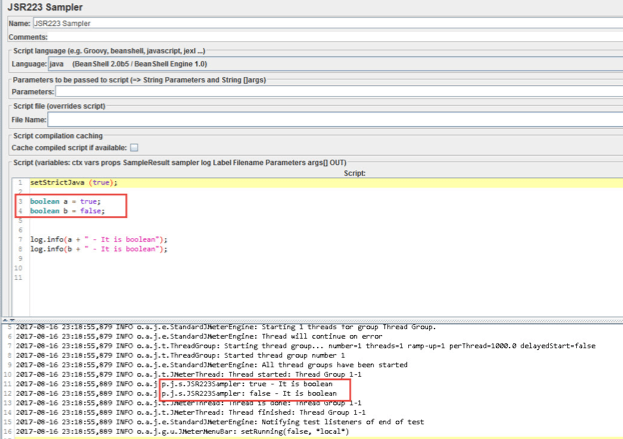This is the example code:

``````setStrictJava (true);

boolean a = true;
boolean b = false;

log.info(a + " - It is boolean");
log.info(b + " - It is boolean");``````

Note: When creating a variable with the type boolean, the value is not enclosed in quotation marks

Variables with a reference data type are variables that reference an object. In Java, all variables whose type is different from a simple type are variables with a reference data type. These are: String, BigInteger, and BigDecimal.

## Variables With a String Data Type (Character Strings)

Variables with a String data type are variables that are an instance of the String class. These variables are immutable. An example of creating variables with a String type is specified in the image below.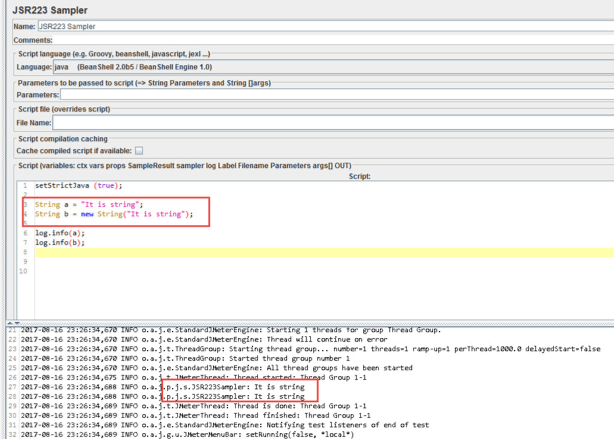This is the example code:

``````setStrictJava (true);

String a = "It is string";
String b = new String("It is string");

log.info(a);
log.info(b);``````

Note: When creating a variable with a String type, the value is in double quotes

## Variables With BigInteger Data Type

Variables with the BigInteger data type are intended for storing integer values of arbitrary length. This type has no restrictions on the allowed values, in contrast to the variables of simple types. The value of a variable of type BigInteger is immutable and can not cause an overflow in arithmetic operations.

An example of creating variables with the type BigInteger is indicated in the image below.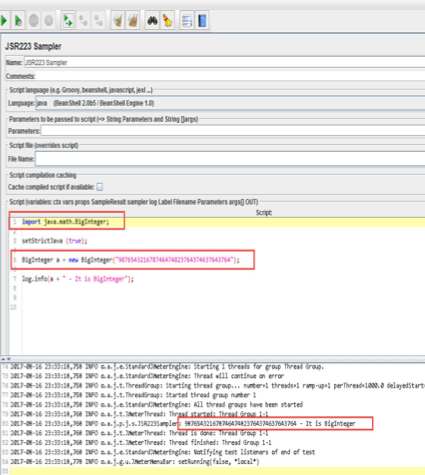This is the example code:

``````import java.math.BigInteger;

setStrictJava (true);

BigInteger a = new BigInteger("987654321678746474823764374637643764");

log.info(a + " - It is BigInteger");``````

## Variables With BigDecimal Type

Variables with BigDecimal data type are intended for storage of floating-point values of arbitrary length. This type has no restrictions on the allowed values, in contrast to the variables of simple types. The value of a variable of type BigDecimal is immutable and can not cause overflows in arithmetic operations. This type is used for financial calculations, in which accuracy is very important. An example of creating variables with the type BigDecimal is shown in the image below.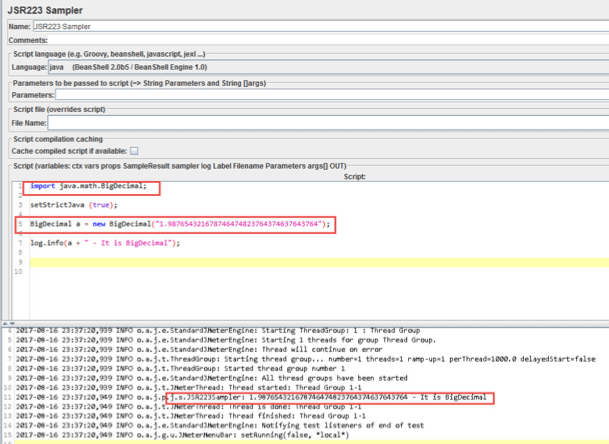This is the example code:

``````import java.math.BigDecimal;

setStrictJava (true);

BigDecimal a = new BigDecimal("1.987654321678746474823764374637643764");

log.info(a + " - It is BigDecimal");``````

In order to work with variables, whether with a simple data type or a reference one, you can use primitive wrappers. Wrappers for primitives are used in different cases. One common example is the use of data structures in tests, which include arrays, lists, collections, and so on. Wrappers are classes that have an analogy with a primitive type, for example, for an int, there is an Integer class. Below is a table of correspondence of a simple type to a similar class.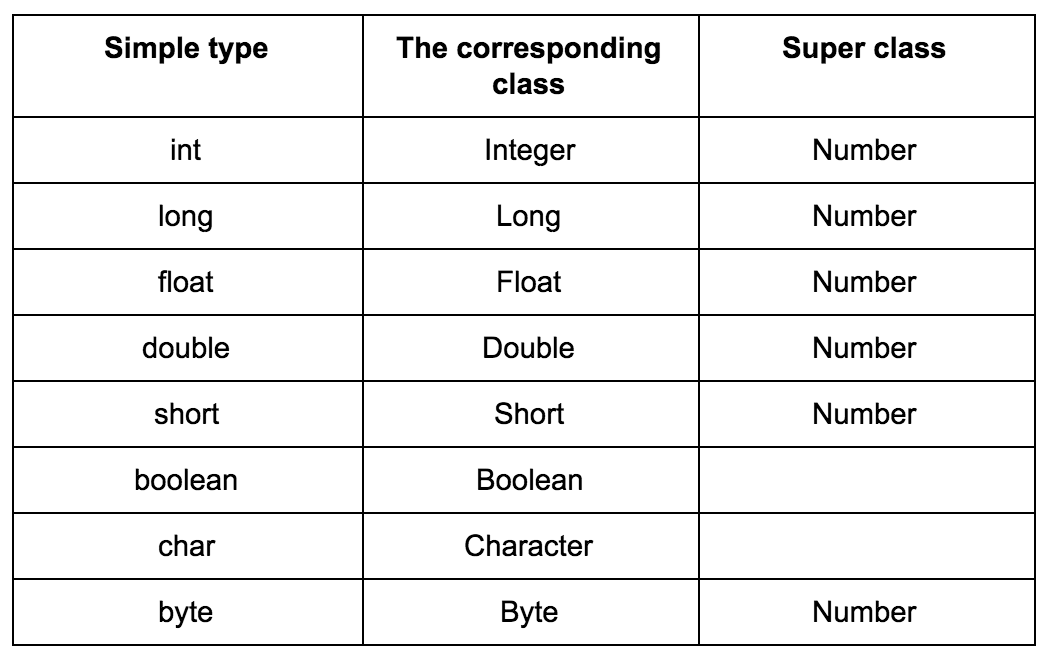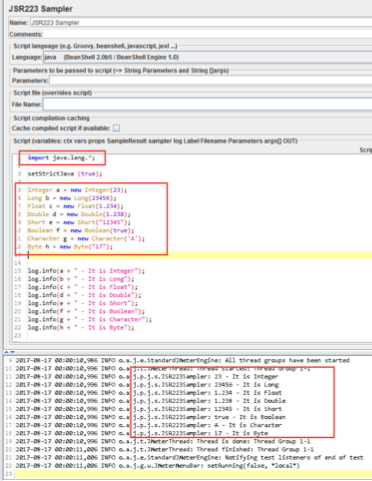This is the example code:

``````import java.lang.*;

setStrictJava (true);

Integer a = new Integer(23);
Long b = new Long(23456);
Float c = new Float(1.234);
Double d = new Double(1.238);
Short e = new Short("12345");
Boolean f = new Boolean(true);
Character g = new Character('A');
Byte h = new Byte("17");

log.info(a + " - It is Integer");
log.info(b + " - It is Long");
log.info(c + " - It is Float");
log.info(d + " - It is Double");
log.info(e + " - It is Short");
log.info(f + " - It is Boolean");
log.info(g + " - It is Character");
log.info(h + " - It is Byte");``````

That's it! You've completed this comprehensive guide to creating variables in JMeter with Java. Stay with us to learn different methods for implementing then, next time.

Topics:
apache jmeter, api testing, beanshell, java, jmeter, load testing, performance

Comment (0)

Save
{{ articles.views | formatCount}} Views

Published at DZone with permission of Sergey Horban , DZone MVB. See the original article here.

Opinions expressed by DZone contributors are their own.